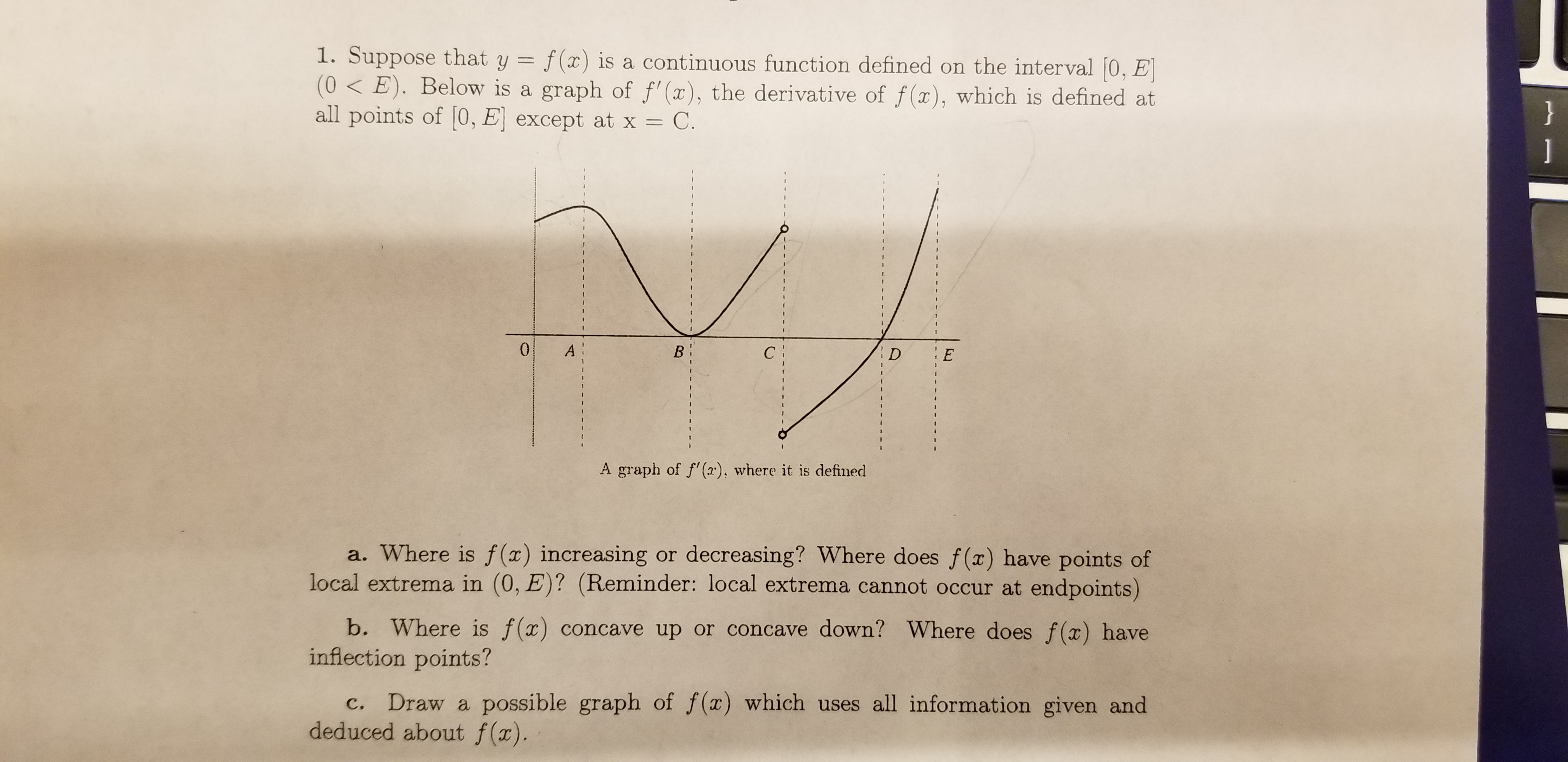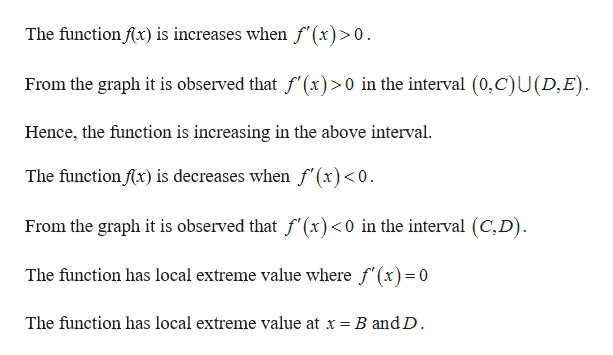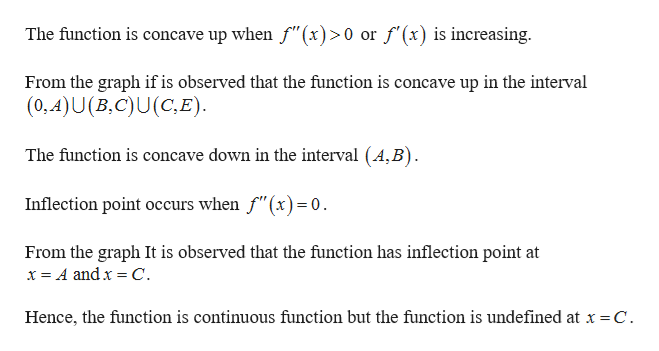# 1. Suppose that y = f(x) is a continuous function defined on the interval 0, E(0 E). Below is a graph of f'(x), the derivative of f(x), which is defined atall points of 0, E except at x =}С.0А!С:EDA graph of f'(), where it is defineda. Where is f (x) increasing or decreasing? Where does f (x) have points oflocal extrema in (0, E)? (Reminder: local extrema cannot occur at endpoints)b. Where is f(x) concave up or concave down? Where does f(x) haveinflection points?Draw a possible graph of f(x) which uses all information given andс.deduced about f(x).B

Question

Question 1 Parts A, B, and Chelp_outlineImage Transcriptionclose1. Suppose that y = f(x) is a continuous function defined on the interval 0, E (0 E). Below is a graph of f'(x), the derivative of f(x), which is defined at all points of 0, E except at x = } С. 0 А! С: E D A graph of f'(), where it is defined a. Where is f (x) increasing or decreasing? Where does f (x) have points of local extrema in (0, E)? (Reminder: local extrema cannot occur at endpoints) b. Where is f(x) concave up or concave down? Where does f(x) have inflection points? Draw a possible graph of f(x) which uses all information given and с. deduced about f(x). B fullscreen
check_circleExpert Solution
Step 1

Consider the given graph.help_outlineImage TranscriptioncloseThe function fx) is increases when f'(x)>0 From the graph it is observed that f'(x)>0 in the interval (0,C)U(D,E) Hence, the function is increasing in the above interval. The function fx) is decreases when f (x)<0 From the graph it is observed that f'(x)<0 in the interval (C,D) The function has local extreme value where f'(x)=0 The function has local extreme value at x = B and D fullscreen
Step 2

(b)

...help_outlineImage TranscriptioncloseThe function is concave up when f"(x)>0 or f'(x) is increasing. From the graph if is observed that the function is concave up in the interval (0,A)U(B,C)U (C,E) The function is concave down in the interval (A, B) Inflection point occurs when f"(x)=0 From the graph It is observed that the function has inflection point at x A andx C. Hence, the function is continuous function but the function is undefined at x = C. fullscreen

### Want to see the full answer?

See Solution

#### Want to see this answer and more?

Solutions are written by subject experts who are available 24/7. Questions are typically answered within 1 hour*

See Solution
*Response times may vary by subject and question
Tagged in

### Other